Anzeige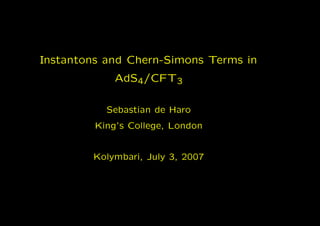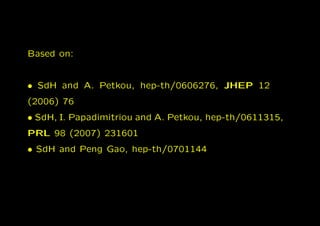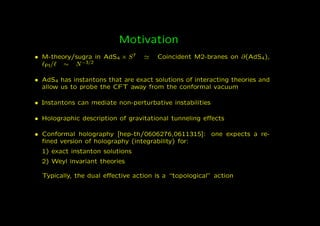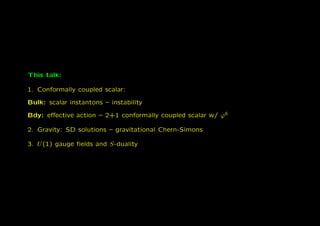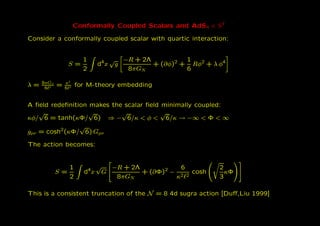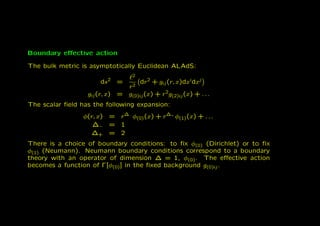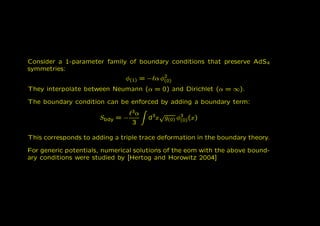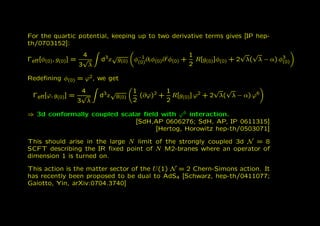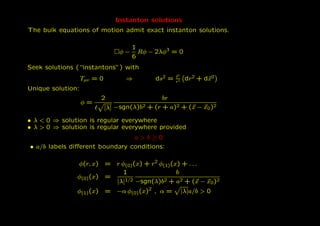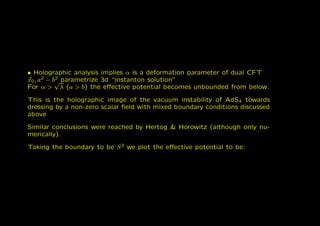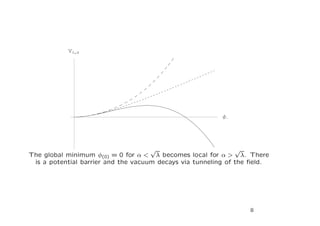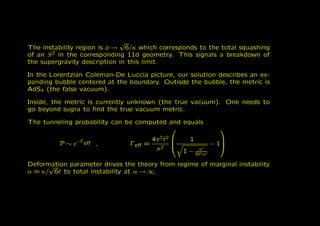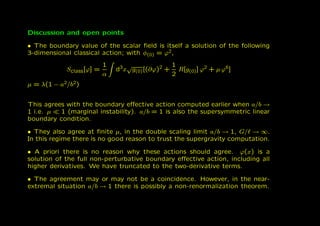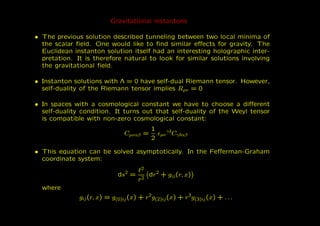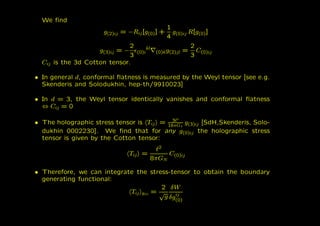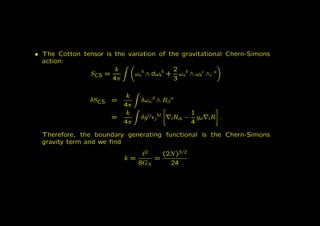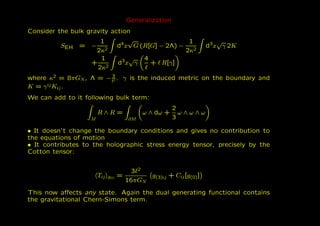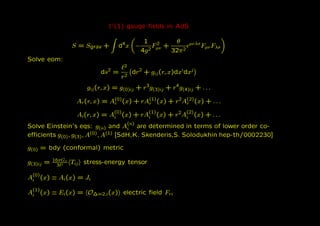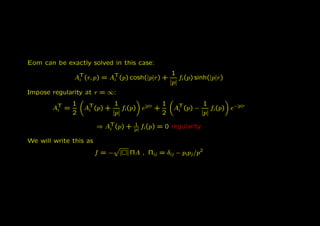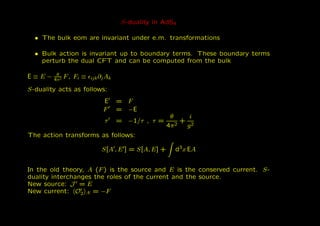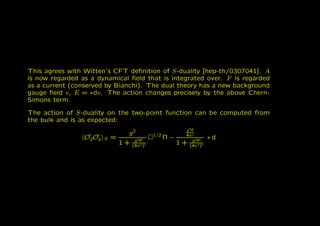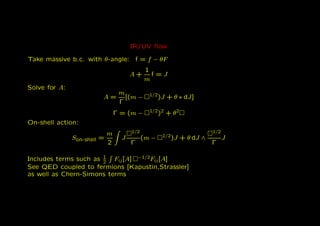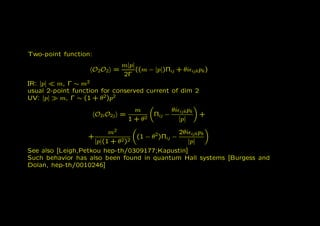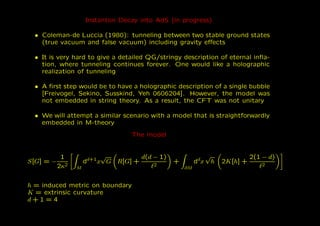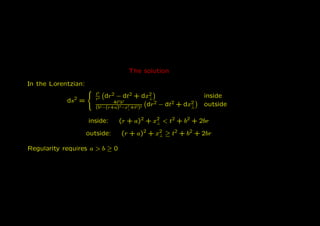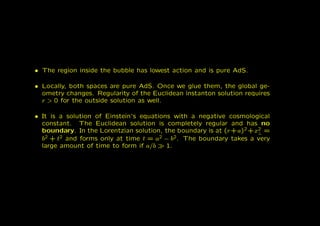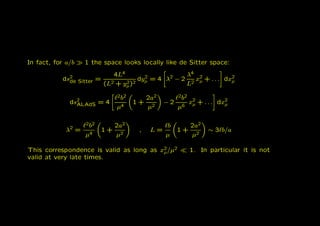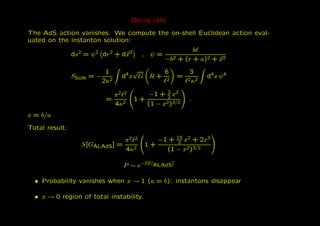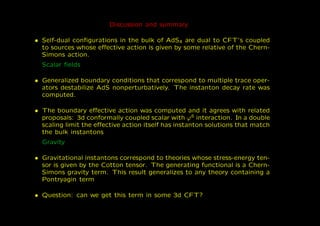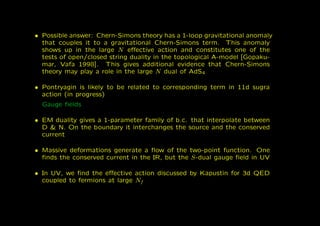1 von 30
Anzeige

### Instantons and Chern-Simons Terms in AdS4/CFT3

1. Instantons and Chern-Simons Terms in AdS4/CFT3 Sebastian de Haro King’s College, London Kolymbari, July 3, 2007
2. Based on: • SdH and A. Petkou, hep-th/0606276, JHEP 12 (2006) 76 • SdH, I. Papadimitriou and A. Petkou, hep-th/0611315, PRL 98 (2007) 231601 • SdH and Peng Gao, hep-th/0701144
3. Motivation • M-theory/sugra in AdS4 × S 7 Coincident M2-branes on ∂(AdS4), Pl/ ∼ N −3/2 • AdS4 has instantons that are exact solutions of interacting theories and allow us to probe the CFT away from the conformal vacuum • Instantons can mediate non-perturbative instabilities • Holographic description of gravitational tunneling eﬀects • Conformal holography [hep-th/0606276,0611315]: one expects a re- ﬁned version of holography (integrability) for: 1) exact instanton solutions 2) Weyl invariant theories Typically, the dual eﬀective action is a “topological” action 1
4. This talk: 1. Conformally coupled scalar: Bulk: scalar instantons – instability Bdy: eﬀective action – 2+1 conformally coupled scalar w/ ϕ6 2. Gravity: SD solutions – gravitational Chern-Simons 3. U (1) gauge ﬁelds and S-duality 2
5. Conformally Coupled Scalars and AdS4 × S 7 Consider a conformally coupled scalar with quartic interaction: 1 √ −R + 2Λ 1 S= d4 x g + (∂φ)2 + Rφ2 + λ φ4 2 8πGN 6 8πGN κ2 λ= 6 2 = 6 2 for M-theory embedding A ﬁeld redeﬁnition makes the scalar ﬁeld minimally coupled: √ √ √ √ κφ/ 6 = tanh(κΦ/ 6) ⇒ − 6/κ < φ < 6/κ → −∞ < Φ < ∞ √ gµν = cosh2(κΦ/ 6) Gµν The action becomes: 1 √ 4 −R + 2Λ 6 2 S= d x G + (∂Φ)2 − 2 2 cosh κΦ 2 8πGN κ 3 This is a consistent truncation of the N = 8 4d sugra action [Duﬀ,Liu 1999] 3
6. Boundary eﬀective action The bulk metric is asymptotically Euclidean ALAdS: 2 2 ds = dr2 + gij (r, x)dxidxj r2 gij (r, x) = g(0)ij (x) + r 2g(2)ij (x) + . . . (1) The scalar ﬁeld has the following expansion: φ(r, x) = r ∆− φ(0) (x) + r ∆+ φ(1) (x) + . . . ∆− = 1 ∆+ = 2 (2) There is a choice of boundary conditions: to ﬁx φ(0) (Dirichlet) or to ﬁx φ(1) (Neumann). Neumann boundary conditions correspond to a boundary theory with an operator of dimension ∆ = 1, φ(0) . The eﬀective action becomes a function of Γ[φ(0) ] in the ﬁxed background g(0)ij . 4
7. Consider a 1-parameter family of boundary conditions that preserve AdS4 symmetries: φ(1) = − α φ2 (0) (3) They interpolate between Neumann (α = 0) and Dirichlet (α = ∞). The boundary condition can be enforced by adding a boundary term: 3α √ Sbdy = − d3 x g(0) φ3 (x) (0) (4) 3 This corresponds to adding a triple trace deformation in the boundary theory. For generic potentials, numerical solutions of the eom with the above bound- ary conditions were studied by [Hertog and Horowitz 2004] 5
8. For the quartic potential, keeping up to two derivative terms gives [IP hep- th/0703152]: 4 √ −1 1 √ √ Γeﬀ[φ(0) , g(0) ] = √ d x g(0) φ(0) ∂i φ(0) ∂ φ(0) + R[g(0) ]φ(0) + 2 λ( λ − α) φ3 3 i (0) 3 λ 2 (5) Redeﬁning φ(0) =ϕ 2 , we get 4 3 √ 1 1 √ √ Γeﬀ[ϕ, g(0) ] = √ d x g(0) (∂ϕ) + R[g(0) ] ϕ + 2 λ( λ − α) ϕ6 2 2 3 λ 2 2 (6) ⇒ 3d conformally coupled scalar ﬁeld with ϕ6 interaction. [SdH,AP 0606276; SdH, AP, IP 0611315] [Hertog, Horowitz hep-th/0503071] This should arise in the large N limit of the strongly coupled 3d N = 8 SCFT describing the IR ﬁxed point of N M2-branes where an operator of dimension 1 is turned on. This action is the matter sector of the U (1) N = 2 Chern-Simons action. It has recently been proposed to be dual to AdS4 [Schwarz, hep-th/0411077; Gaiotto, Yin, arXiv:0704.3740] 6
9. Instanton solutions The bulk equations of motion admit exact instanton solutions. 1 φ− Rφ − 2λφ3 = 0 (7) 6 Seek solutions (“instantons”) with 2 Tµν = 0 ⇒ ds2 = r2 dr2 + dx2 Unique solution: 2 br φ= (8) |λ| −sgn(λ)b2 + (r + a)2 + (x − x0 )2 • λ < 0 ⇒ solution is regular everywhere • λ > 0 ⇒ solution is regular everywhere provided a>b≥0 • a/b labels diﬀerent boundary conditions: φ(r, x) = r φ(0) (x) + r 2 φ(1) (x) + . . . (9) 1 b φ(0) (x) = (10) |λ|1/2 −sgn(λ)b2 + a2 + (x − x0 )2 φ(1) (x) = −α φ(0) (x)2 , α = |λ|a/b > 0 (11) (12) 7
10. • Holographic analysis implies α is a deformation parameter of dual CFT x0 , a2 − b√ parametrize 3d “instanton solution” 2 For α > λ (a > b) the eﬀective potential becomes unbounded from below. This is the holographic image of the vacuum instability of AdS4 towards dressing by a non-zero scalar ﬁeld with mixed boundary conditions discussed above Similar conclusions were reached by Hertog & Horowitz (although only nu- merically). Taking the boundary to be S 3 we plot the eﬀective potential to be:
11. VΛ,Α Φ √ √ The global minimum φ(0) = 0 for α < λ becomes local for α > λ. There is a potential barrier and the vacuum decays via tunneling of the ﬁeld. 8
12. √ The instability region is φ → 6/κ which corresponds to the total squashing of an S 2 in the corresponding 11d geometry. This signals a breakdown of the supergravity description in this limit. In the Lorentzian Coleman-De Luccia picture, our solution describes an ex- panding bubble centered at the boundary. Outisde the bubble, the metric is AdS4 (the false vacuum). Inside, the metric is currently unknown (the true vacuum). One needs to go beyond sugra to ﬁnd the true vacuum metric. The tunneling probability can be computed and equals   −Γeﬀ 4π 2 2 1 P ∼e , Γeﬀ = − 1 (13)   κ2  2 κ 1− 6 2 α2 Deformation parameter drives the theory from regime of marginal instability √ α = κ/ 6 to total instability at α → ∞. 9
13. Discussion and open points • The boundary value of the scalar ﬁeld is itself a solution of the following 3-dimensional classical action; with φ(0) = ϕ2 , 1 √ 1 Sclass[ϕ] = d3x g(0) [(∂ϕ)2 + R[g(0) ] ϕ2 + µ ϕ6 ] α 2 µ = λ(1 − a2/b2) This agrees with the boundary eﬀective action computed earlier when a/b → 1 i.e. µ 1 (marginal instability). a/b = 1 is also the supersymmetric linear boundary condition. • They also agree at ﬁnite µ, in the double scaling limit a/b → 1, G/ → ∞. In this regime there is no good reason to trust the supergravity computation. • A priori there is no reason why these actions should agree. ϕ(x) is a solution of the full non-perturbative boundary eﬀective action, including all higher derivatives. We have truncated to the two-derivative terms. • The agreement may or may not be a coincidence. However, in the near- extremal situation a/b → 1 there is possibly a non-renormalization theorem. 10
14. Gravitational instantons • The previous solution described tunneling between two local minima of the scalar ﬁeld. One would like to ﬁnd similar eﬀects for gravity. The Euclidean instanton solution itself had an interesting holographic inter- pretation. It is therefore natural to look for similar solutions involving the gravitational ﬁeld. • Instanton solutions with Λ = 0 have self-dual Riemann tensor. However, self-duality of the Riemann tensor implies Rµν = 0 • In spaces with a cosmological constant we have to choose a diﬀerent self-duality condition. It turns out that self-duality of the Weyl tensor is compatible with non-zero cosmological constant: 1 γδ Cµναβ = µν Cγδαβ 2 • This equation can be solved asymptotically. In the Feﬀerman-Graham coordinate system: 2 2 ds = dr2 + gij (r, x) r2 where gij (r, x) = g(0)ij (x) + r 2g(2)ij (x) + r 3g(3)ij (x) + . . . 11
15. We ﬁnd 1 g(2)ij = −Rij [g(0) ] + g(0)ij R[g(0) ] 4 2 kl 2 g(3)ij = − (0)i (0)k g(2)jl = C(0)ij 3 3 Cij is the 3d Cotton tensor. • In general d, conformal ﬂatness is measured by the Weyl tensor [see e.g. Skenderis and Solodukhin, hep-th/9910023] • In d = 3, the Weyl tensor identically vanishes and conformal ﬂatness ⇔ Cij = 0 2 3 • The holographic stress tensor is Tij = 16πGN g(3)ij [SdH,Skenderis, Solo- dukhin 0002230]. We ﬁnd that for any g(0)ij the holographic stress tensor is given by the Cotton tensor: 2 Tij = C(0)ij 8πGN • Therefore, we can integrate the stress-tensor to obtain the boundary generating functional: 2 δW Tij g(0) = √ ij g δg(0)
16. • The Cotton tensor is the variation of the gravitational Chern-Simons action: k 2 SCS = ωab ∧ dωbb + ωab ∧ ωb c ∧c a 4π 3 k δSCS = δωαβ ∧ Rβ α 4π k 1 = δg ij j kl l Rik − gil lR . (14) 4π 4 Therefore, the boundary generating functional is the Chern-Simons gravity term and we ﬁnd 2 (2N )3/2 k= = 8GN 24
17. Generalization Consider the bulk gravity action 1 4 √ 1 √ SEH = − 2 d x G (R[G] − 2Λ) − d3x γ 2K 2κ 2κ2 1 √ 4 + 2 d3 x γ + R[γ] (15) 2κ where κ2 = 8πGN , Λ = − 3 . γ is the induced metric on the boundary and 2 K=γ ij K . ij We can add to it following bulk term: 2 R∧R = ω ∧ dω + ω∧ω∧ω M ∂M 3 • It doesn’t change the boundary conditions and gives no contribution to the equations of motion • It contributes to the holographic stress energy tensor, precisely by the Cotton tensor: 3 2 Tij g(0) = g(3)ij + Cij [g(0) ] 16πGN This now aﬀects any state. Again the dual generating functional contains the gravitational Chern-Simons term. 12
18. U (1) gauge ﬁelds in AdS 1 2 θ S = Sgrav + d4 x − Fµν + µνλσ Fµν Fλσ 4g 2 32π 2 Solve eom: 2 2 ds = dr2 + gij (r, x)dxidxj r2 gij (r, x) = g(0)ij + r 3g(3)ij + r 4g(4)ij + . . . Ar (r, x) = A(0) (x) + rA(1) (x) + r 2A(2) (x) + . . . r r r Ai(r, x) = A(0) (x) + rA(1) (x) + r 2 A(2) (x) + . . . i i i Solve Einstein’s eqs: g(n) and A(n) are determined in terms of lower order co- i eﬃcients g(0) , g(3) , A (0) , A(1) [SdH,K. Skenderis,S. Solodukhin hep-th/0002230] g(0) = bdy (conformal) metric 16πGN g(3)ij = 3 2 Tij stress-energy tensor A(0) (x) ≡ Ai(x) = Ji i A(1) (x) ≡ Ei (x) = O∆=2,i (x) electric ﬁeld Fri i 13
19. Eom can be exactly solved in this case: 1 AT(r, p) = AT(p) cosh(|p|r) + i i fi (p) sinh(|p|r) |p| Impose regularity at r = ∞: 1 1 1 1 AT = i AT(p) + i fi(p) e|p|r + AT(p) − i fi(p) e−|p|r 2 |p| 2 |p| ⇒ AT(p) + i 1 |p| fi (p) = 0 regularity We will write this as f =− | | ΠA , Πij = δij − pipj /p2 14
20. S-duality in AdS4 • The bulk eom are invariant under e.m. transformations • Bulk action is invariant up to boundary terms. These boundary terms perturb the dual CFT and can be computed from the bulk θ E≡E− 4π 2 F , Fi ≡ ijk ∂j Ak S-duality acts as follows: E = F F = −E θ i τ = −1/τ , τ = + 2 (16) 4π 2 g The action transforms as follows: S[A , E ] = S[A, E] + d 3 x EA In the old theory, A (F ) is the source and E is the conserved current. S- duality interchanges the roles of the current and the source. New source: J = E New current: O2 A = −F 15
21. This agrees with Witten’s CFT deﬁnition of S-duality [hep-th/0307041]. A is now regarded as a dynamical ﬁeld that is integrated over. F is regarded as a current (conserved by Bianchi). The dual theory has a new background gauge ﬁeld v, E = ∗dv. The action changes precisely by the above Chern- Simons term. The action of S-duality on the two-point function can be computed from the bulk and is as expected: g4θ g2 1/2 4π 2 O 2 O2 A = g θ Π− ∗d + (4πθ2)2 g4 2 4 2 1+ (4π 2 )2 1
22. IR/UV ﬂow Take massive b.c. with θ-angle: f = f − θF 1 A+ f=J m Solve for A: m 1/2 A= [(m − )J + θ ∗ dJ] Γ 1/2 2 Γ = (m − ) + θ2 On-shell action: m 1/2 1/2 1/2 Son-shell = J (m − )J + θ dJ ∧ J 2 Γ Γ Includes terms such as 1 Fij [A] −1/2 Fij [A] 2 See QED coupled to fermions [Kapustin,Strassler] as well as Chern-Simons terms 16
23. Two-point function: m|p| O 2 O2 = ((m − |p|)Πij + θi ijk pk ) 2Γ IR: |p| m, Γ ∼ m2 usual 2-point function for conserved current of dim 2 UV: |p| m, Γ ∼ (1 + θ 2)p2 m θi ijk pk O2i O2j = Πij − + 1 + θ2 |p| m2 2θi ijk pk + (1 − θ 2 )Πij − |p|(1 + θ 2 )2 |p| See also [Leigh,Petkou hep-th/0309177;Kapustin] Such behavior has also been found in quantum Hall systems [Burgess and Dolan, hep-th/0010246] 17
24. Instanton Decay into AdS (in progress) • Coleman-de Luccia (1980): tunneling between two stable ground states (true vacuum and false vacuum) including gravity eﬀects • It is very hard to give a detailed QG/stringy description of eternal inﬂa- tion, where tunneling continues forever. One would like a holographic realization of tunneling • A ﬁrst step would be to have a holographic description of a single bubble [Freivogel, Sekino, Susskind, Yeh 0606204]. However, the model was not embedded in string theory. As a result, the CFT was not unitary • We will attempt a similar scenario with a model that is straightforwardly embedded in M-theory The model 1 d+1 √ d(d − 1) d √ 2(1 − d) S[G] = − 2 d x G R[G] + 2 + d x h 2K[h] + 2 2κ M ∂M (17) h = induced metric on boundary K = extrinsic curvature d+1=4 18
25. The solution In the Lorentzian: 2 2 r 2 dr2 − dt2 + dx2 ⊥ inside ds = 4 2 b2 (b2 −(r+a)2 −x2 +t2 )2 dr2 − dt2 + dx2 ⊥ outside ⊥ inside: (r + a)2 + x2 < t2 + b2 + 2br ⊥ outside: (r + a)2 + x2 ≥ t2 + b2 + 2br ⊥ Regularity requires a > b ≥ 0 19
26. • The region inside the bubble has lowest action and is pure AdS. • Locally, both spaces are pure AdS. Once we glue them, the global ge- ometry changes. Regularity of the Euclidean instanton solution requires r > 0 for the outside solution as well. • It is a solution of Einstein’s equations with a negative cosmological constant. The Euclidean solution is completely regular and has no boundary. In the Lorentzian solution, the boundary is at (r +a)2 +x2 = ⊥ b 2 + t2 and forms only at time t = a2 − b2 . The boundary takes a very large amount of time to form if a/b 1. 21
27. In fact, for a/b 1 the space looks locally like de Sitter space: 2 4L4 λ4 2 dsde Sitter = dyµ = 4 λ − 2 2 xµ + . . . dx2 2 2 µ (L2 + yµ )2 2 L 2 b2 2a2 2 b2 2 dsALAdS = 4 1+ 2 −2 x2 + . . . dx2 µ µ µ4 µ µ6 2 b2 2a2 b 2a2 2 λ = 1+ 2 , L= 1+ 2 ∼ 3 b/a µ4 µ µ µ This correspondence is valid as long as x2 /µ2 µ 1. In particular it is not valid at very late times. 22
28. Decay rate The AdS action vanishes. We compute the on-shell Euclidean action eval- uated on the instanton solution: b ds2 = ψ 2 dr2 + dx2 , ψ= −b2 + (r + a)2 + x2 1 4 √ 6 3 Sbulk = − 2 d x G R+ 2 = 2 κ2 d4 x ψ 4 2κ 3 2 π2 2 −1 + 2 x = 1+ . 4κ2 (1 − x2 )3/2 x = b/a Total result: 15 π2 2 −1 + 2 x2 + 2x3 S[GALAdS ] = 1+ 4κ2 (1 − x2)3/2 P ∼ e−S[GALAdS ] • Probability vanishes when x → 1 (a = b): instantons disappear • x → 0 region of total instability. 23
29. Discussion and summary • Self-dual conﬁgurations in the bulk of AdS4 are dual to CFT’s coupled to sources whose eﬀective action is given by some relative of the Chern- Simons action. Scalar ﬁelds • Generalized boundary conditions that correspond to multiple trace oper- ators destabilize AdS nonperturbatively. The instanton decay rate was computed. • The boundary eﬀective action was computed and it agrees with related proposals: 3d conformally coupled scalar with ϕ6 interaction. In a double scaling limit the eﬀective action itself has instanton solutions that match the bulk instantons Gravity • Gravitational instantons correspond to theories whose stress-energy ten- sor is given by the Cotton tensor. The generating functional is a Chern- Simons gravity term. This result generalizes to any theory containing a Pontryagin term • Question: can we get this term in some 3d CFT? 24
30. • Possible answer: Chern-Simons theory has a 1-loop gravitational anomaly that couples it to a gravitational Chern-Simons term. This anomaly shows up in the large N eﬀective action and constitutes one of the tests of open/closed string duality in the topological A-model [Gopaku- mar, Vafa 1998]. This gives additional evidence that Chern-Simons theory may play a role in the large N dual of AdS4 • Pontryagin is likely to be related to corresponding term in 11d sugra action (in progress) Gauge ﬁelds • EM duality gives a 1-parameter family of b.c. that interpolate between D & N. On the boundary it interchanges the source and the conserved current • Massive deformations generate a ﬂow of the two-point function. One ﬁnds the conserved current in the IR, but the S-dual gauge ﬁeld in UV • In UV, we ﬁnd the eﬀective action discussed by Kapustin for 3d QED coupled to fermions at large Nf
Anzeige